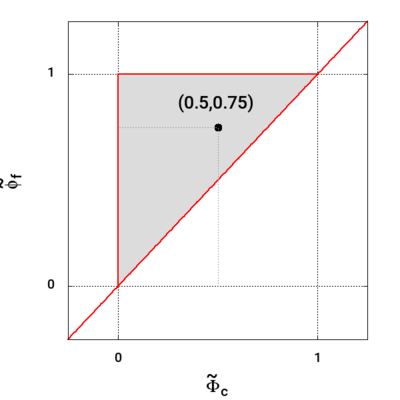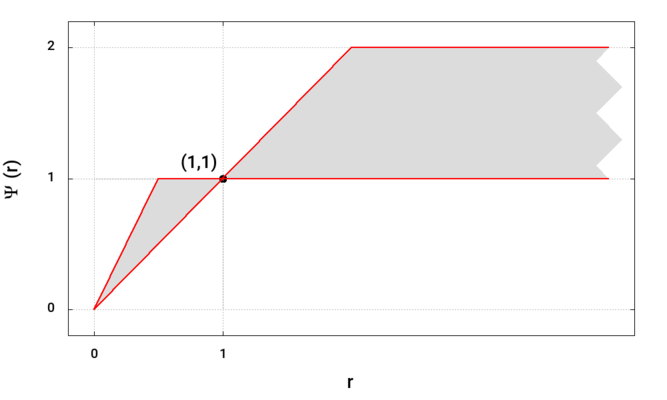Many of the convection schemes in OpenFOAM are based on the Normalised Variable (NV)  and Total Variation Diminishing (TVD)  methods. These provide a set tools to characterise scheme properties such as boundedness (1-D).## Properties🔗

• Offer a blend between a low order scheme and a higher order scheme based on the calculation of a limiter
• Boundedness of NVD/TVD schemes is only guaranteed for 1-D cases
• Boundedness can be improved in 2-D and 3-D cases by limiting the gradient

## Limiter calculation🔗

For arbitrary unstructured meshes it is not straightforward to identify the upstream and downstream locations reliably. The limiters are evaluated using a compact stencil as described in Jasak et al. , where:

TVD: Limiter $$\Psi(r)$$ defined as a function of $$r$$ $$r = 2 \frac{\vec{d} \dprod \left( \grad{\phi} \right)_P}{\vec{d} \dprod \left(\grad{\phi} \right)_f} - 1 = 2 \frac{\vec{d} \dprod \left( \grad{\phi} \right)_P}{\phi_N - \phi_P} - 1$$ NVD: Limiter $$\widetilde{\phi_f}$$ defined as a function of $$\widetilde{\phi_c}$$ $$\widetilde{\phi_c} = 1 - 0.5 \frac{\vec{d} \dprod \left( \grad{\phi} \right)_f}{\vec{d} \dprod \left( \grad{\phi}\right)_P } = 1 - 0.5 \frac{\phi_N - \phi_P}{\vec{d} \dprod \left(\grad{\phi}\right)_P}$$ Where the gradient at cell P $$\left( \grad{\phi} \right)_P$$ is calculated using the user-selected gradient scheme, and the vector $$\vec{d}$$ $$\vec{d} = \vec{C}_{N} - \vec{C}_{P}$$

Source code:

API: